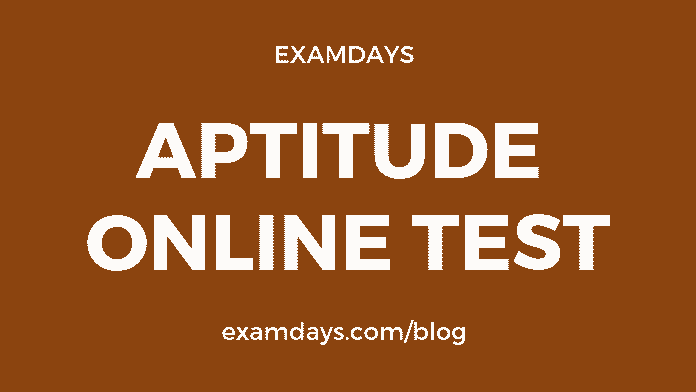Aptitude Online Test Quiz #8 with Answers

0Aptitude Online Test

General quantitative aptitude online test is more important for the various examinations, such as SSC, Railways RRB, PSU, State PSC, IBPS Banks, and other Government notifications, The aptitude questions practice make sense in the examination purpose, candidates should practice as many of questions and different models of questions.

Q1. Find the greatest number, which on dividing 1657 and 2037 leaves remainders 6 and 5 respectively.?

1. 127
2. 132
3. 145
4. 175

127

Q2. The traffic lights at three different road crossings change after every 40 sec, 72 sec and 108 sec respectively. If they all change simultaneously at 5: 20:00 hours, then find the time at which they will change simultaneously. ?

1. 5 : 28 : 00 hrs
2. 5 : 30 : 00 hrs
3. 5 : 38 : 00 hrs
4. 5 : 40 : 00 hrs

5 : 30 : 00 hrs

Q3. Ram got married to Sita 10 years ago. Today his age is 7/5 times of his age at the time of his marriage. Find his age at the time of marriage.?

1. 22 Years
2. 24 Years
3. 25 Years
4. 30 Years

25 Years

Q4. Find the compound interest on a principal amount of Rs.5000 after 2 years, if the rate of interest for the 1st year is 2% and for the 2nd year is 4%.?

1. 104
2. 204
3. 304
4. 404

304

Q5. What will be the amount of the sum of Rs.10,00,000 is invested at compound interest for 3 years with a rate of interest 11%, 12% and 13% respectively?

1. Rs.12,14,816
2. Rs.14,04,816
3. Rs.16,00,816
4. Rs.11,35,816

Rs.14,04,816

Q6. P, Q and R are in a cycle race of 4500 meters. P cycles twice as fast as Q. R cycles 1/3rd as fast as Q. R completes the race in 45 minutes. Then there was Q from the finishing line when P finished the race?

1. 300m
2. 1500m
3. 2250m
4. 3000m

2250m

Q7. A walks from points Jammu to Delhi and at the same time, B starts walking from Delhi to Jammu. After passing each other, they complete their journeys in 361 hours and 289 hours, respectively. Find the ratio of the speed of A to that of B?

1. 17:19
2. 289:361
3. 361:289
4. 19:17
1. 17:19

Q8. Equal weights of two alloys containing tin, copper and lead in the ratio 3:2:7 and 4:11:3 are melted and mixed together. What is the ratio of tin, copper and lead in the resultant alloy?

1. 41:81:37
2. 33:91:81
3. 17:28:27
4. 51:86:89

17:28:27

Q9. The average mark of a class of twenty students is 64. If three students whose marks are 32,28 and 34 are removed, then find the approximate average mark of the remaining students of the class.?

1. 71
2. 74
3. 57
4. 70

70

Q10. Find the average of all the numbers between 6 and 34 which are divisible by 5?

1. 18
2. 20
3. 24
4. 30